Chapter 9 Stoichiometry Stoichiometry Composition Stoichiometry deals with

• Slides: 23Chapter 9 StoichiometryStoichiometry Composition Stoichiometry: deals with the mass relationships of elements in compounds. Reaction Stoichiometry: involves mass relationships between reactants and products in a chemical rxn. Ch. 9 -2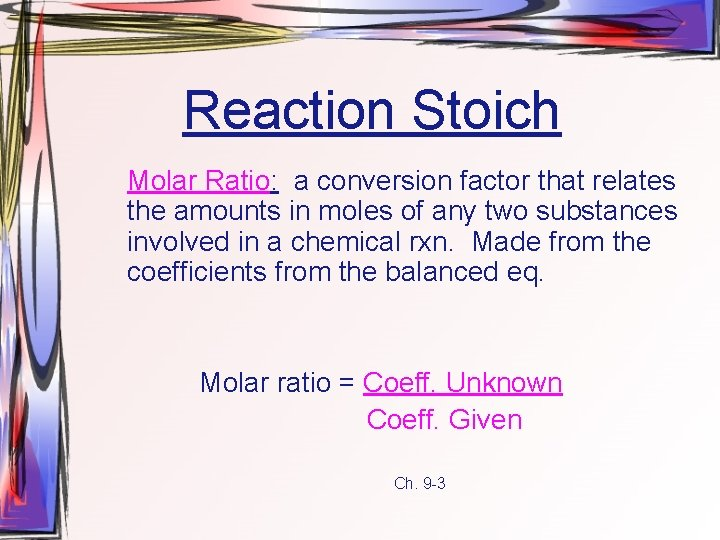Reaction Stoich Molar Ratio: a conversion factor that relates the amounts in moles of any two substances involved in a chemical rxn. Made from the coefficients from the balanced eq. Molar ratio = Coeff. Unknown Coeff. Given Ch. 9 -3Type 1 Mole to Mole Ex. How many moles of Al(NO 3)3 are produced when 0. 75 mol of Ag. NO 3 react in this equation? 3 Ag. NO 3 + Al → Al(NO 3)3 + 3 Ag 0. 75 mol Ag. NO 3 x 1 mol Al(NO 3)3 = 0. 25 mol Al(NO 3)3 3 mol Ag. NO 3 Ch. 9 -4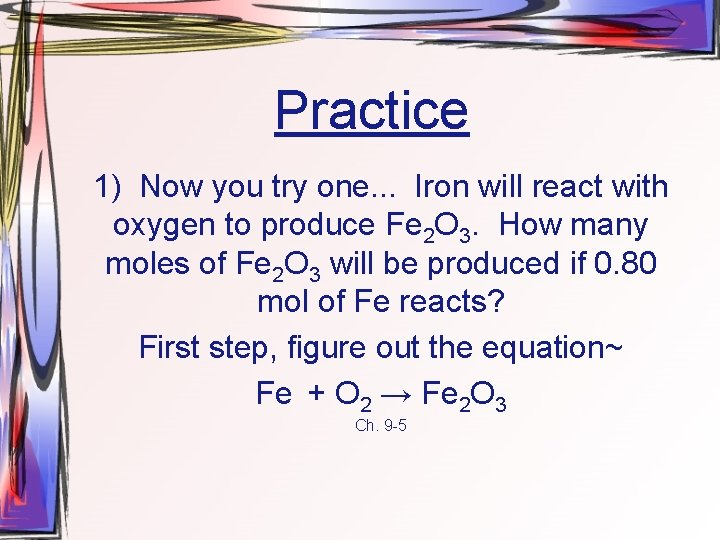Practice 1) Now you try one. . . Iron will react with oxygen to produce Fe 2 O 3. How many moles of Fe 2 O 3 will be produced if 0. 80 mol of Fe reacts? First step, figure out the equation~ Fe + O 2 → Fe 2 O 3 Ch. 9 -5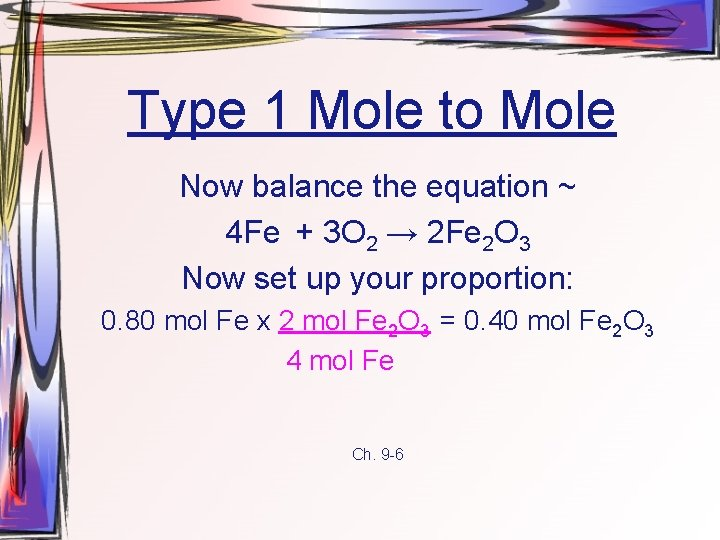Type 1 Mole to Mole Now balance the equation ~ 4 Fe + 3 O 2 → 2 Fe 2 O 3 Now set up your proportion: 0. 80 mol Fe x 2 mol Fe 2 O 3 = 0. 40 mol Fe 2 O 3 4 mol Fe Ch. 9 -6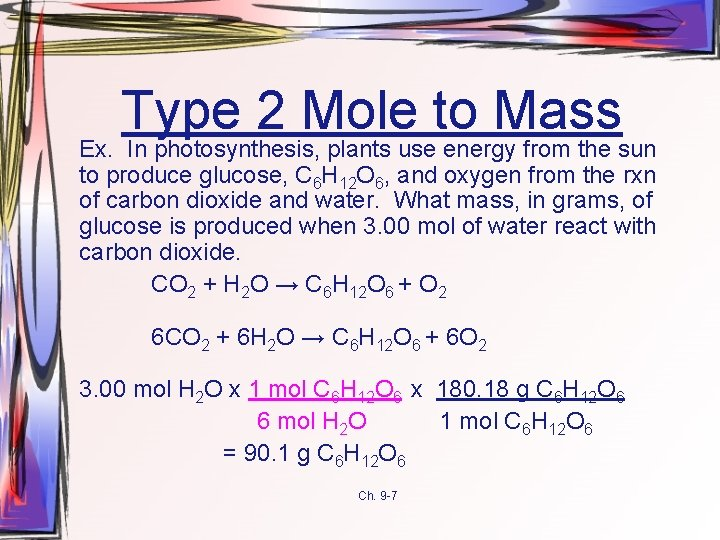Type 2 Mole to Mass Ex. In photosynthesis, plants use energy from the sun to produce glucose, C 6 H 12 O 6, and oxygen from the rxn of carbon dioxide and water. What mass, in grams, of glucose is produced when 3. 00 mol of water react with carbon dioxide. CO 2 + H 2 O → C 6 H 12 O 6 + O 2 6 CO 2 + 6 H 2 O → C 6 H 12 O 6 + 6 O 2 3. 00 mol H 2 O x 1 mol C 6 H 12 O 6 x 180. 18 g C 6 H 12 O 6 6 mol H 2 O 1 mol C 6 H 12 O 6 = 90. 1 g C 6 H 12 O 6 Ch. 9 -7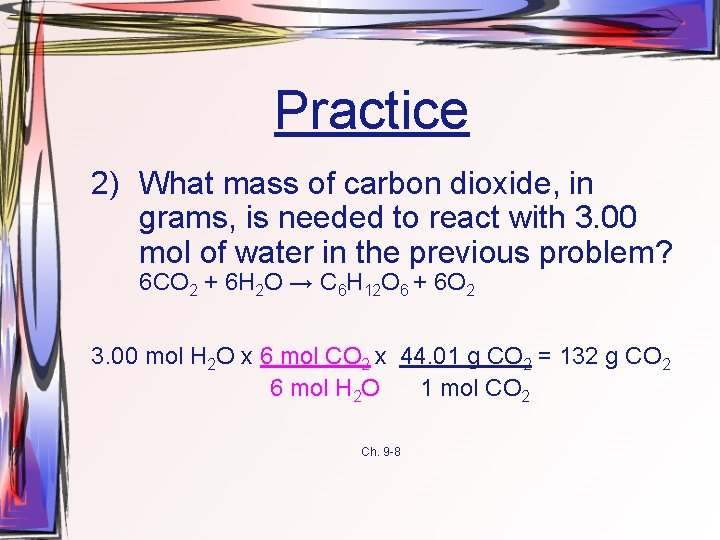Practice 2) What mass of carbon dioxide, in grams, is needed to react with 3. 00 mol of water in the previous problem? 6 CO 2 + 6 H 2 O → C 6 H 12 O 6 + 6 O 2 3. 00 mol H 2 O x 6 mol CO 2 x 44. 01 g CO 2 = 132 g CO 2 6 mol H 2 O 1 mol CO 2 Ch. 9 -8Type 3 Mass to Moles Ex. The first step in the industrial manufacture of nitric acid is the catalytic oxidation of ammonia, ammonia reacts with oxygen to produce nitrogen monoxide and water. If the rxn is run using 824 g of ammonia and excess oxygen, how many moles of water are formed? NH 3 + O 2 → NO + H 2 O 4 NH 3 + 5 O 2 → 4 NO + 6 H 2 O 824 g NH 3 x 1 mol NH 3 x 6 mol H 2 O = 72. 5 mol H 2 O 17. 04 g NH 3 4 mol NH 3 Ch. 9 -9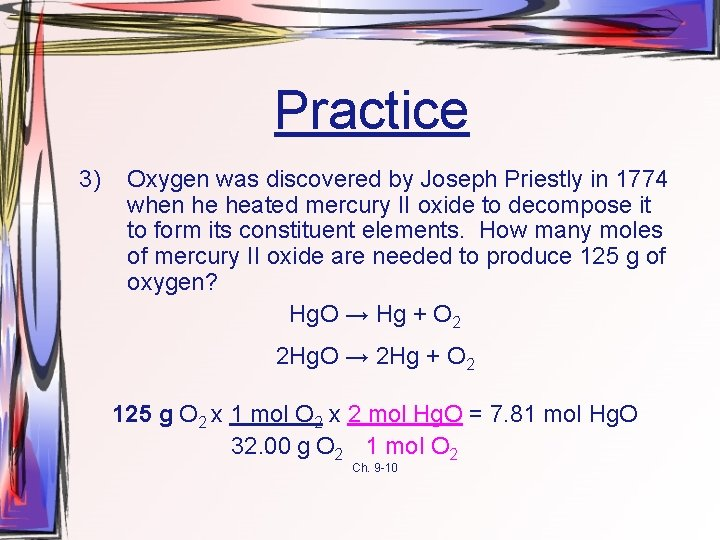Practice 3) Oxygen was discovered by Joseph Priestly in 1774 when he heated mercury II oxide to decompose it to form its constituent elements. How many moles of mercury II oxide are needed to produce 125 g of oxygen? Hg. O → Hg + O 2 2 Hg. O → 2 Hg + O 2 125 g O 2 x 1 mol O 2 x 2 mol Hg. O = 7. 81 mol Hg. O 32. 00 g O 2 1 mol O 2 Ch. 9 -10Type 4 Mass to Mass Ex. How much Li. OH is produced when 0. 38 g of Li 3 N reacts with H 2 O? Ammonia is also produced. Li 3 N + H 2 O → Li. OH + NH 3 Li 3 N + 3 H 2 O → 3 Li. OH + NH 3 0. 38 g. Li 3 N x 1 mol Li 3 N x 3 mol Li. OH x 23. 94 g Li. OH 34. 83 g Li 3 N 1 mol. Li 3 N 1 mol Li. OH = 0. 78 g Li. OH Ch. 9 -11Practice 4) Now you try one: What mass of sodium chloride is produced when chlorine reacts with 0. 30 g of sodium iodide? Na. I + Cl 2 → I 2 + Na. Cl 2 Na. I + Cl 2 → I 2 + 2 Na. Cl 0. 30 g. Na. I x 1 mol Na. I x 2 mol Na. Cl x 58. 44 g Na. Cl 149. 89 g Na. I 2 mol Na. I 1 mol Na. Cl = 0. 12 g Na. Cl Ch. 9 -12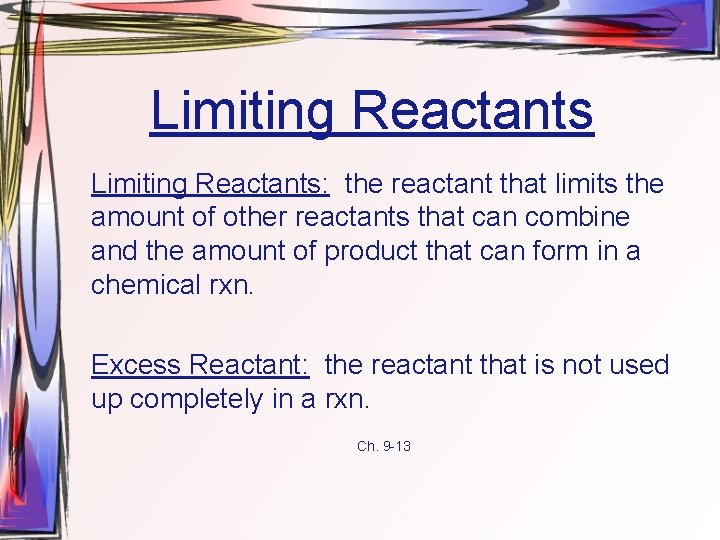Limiting Reactants: the reactant that limits the amount of other reactants that can combine and the amount of product that can form in a chemical rxn. Excess Reactant: the reactant that is not used up completely in a rxn. Ch. 9 -13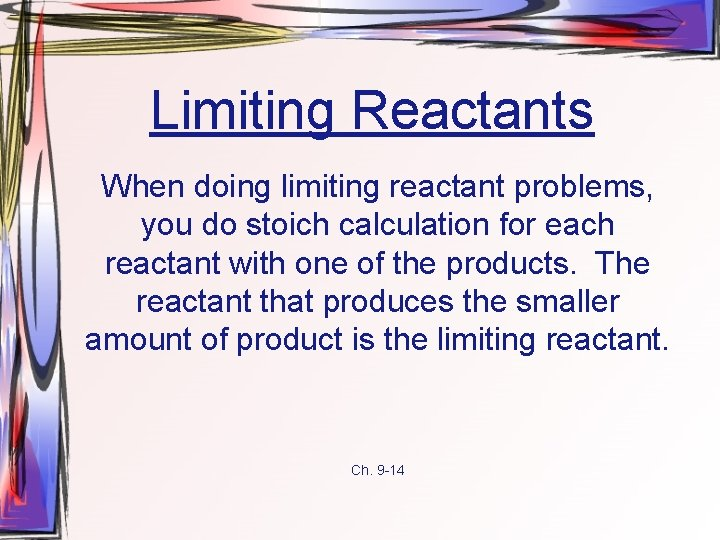Limiting Reactants When doing limiting reactant problems, you do stoich calculation for each reactant with one of the products. The reactant that produces the smaller amount of product is the limiting reactant. Ch. 9 -14Limiting Reactants Ex. Zinc and sulfur react to form zinc sulfide according to the following rxn: 8 Zn + S 8 → 8 Zn. S a) If 2. 00 mol Zn are heated with 1. 00 mol S 8, identify the limiting reactant. b) How many moles of excess reactant remain? c) How many moles of product are formed? a) 2. 00 mol Zn x 8 mol Zn. S = 2. 00 mol Zn. S 8 mol Zn 1. 00 mol S 8 x 8 mol Zn. S = 8. 00 mol Zn. S 1 mol S 8 9 -15 Zn is the limiting reactant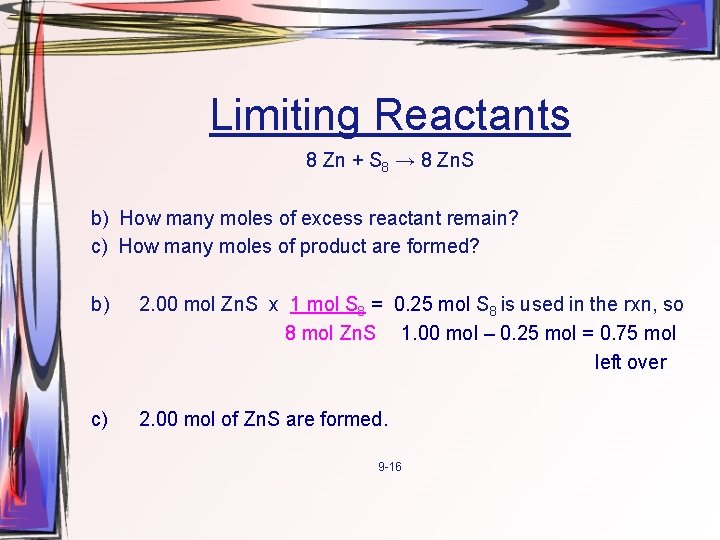Limiting Reactants 8 Zn + S 8 → 8 Zn. S b) How many moles of excess reactant remain? c) How many moles of product are formed? b) 2. 00 mol Zn. S x 1 mol S 8 = 0. 25 mol S 8 is used in the rxn, so 8 mol Zn. S 1. 00 mol – 0. 25 mol = 0. 75 mol left over c) 2. 00 mol of Zn. S are formed. 9 -16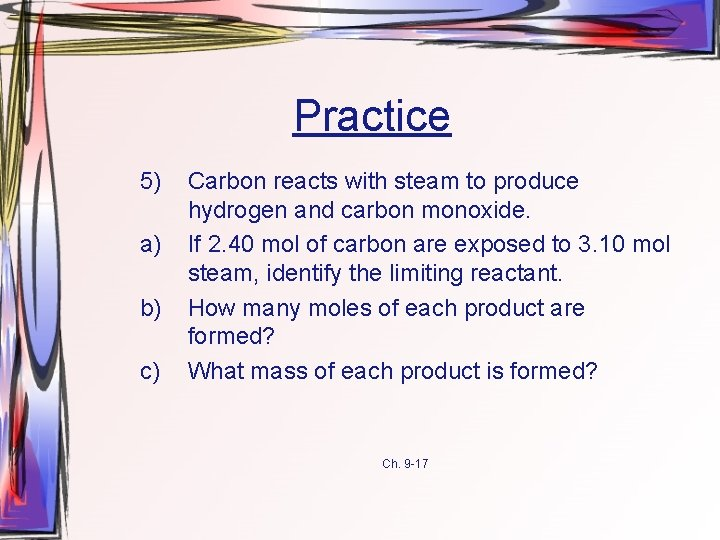Practice 5) a) b) c) Carbon reacts with steam to produce hydrogen and carbon monoxide. If 2. 40 mol of carbon are exposed to 3. 10 mol steam, identify the limiting reactant. How many moles of each product are formed? What mass of each product is formed? Ch. 9 -17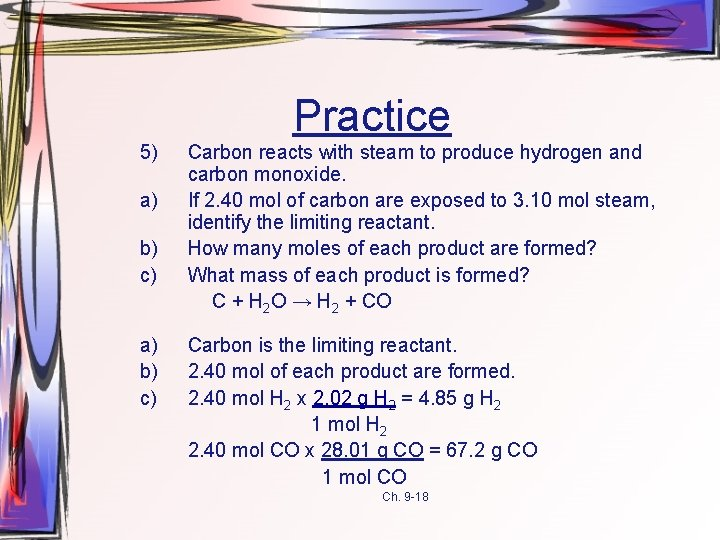Practice 5) a) b) c) Carbon reacts with steam to produce hydrogen and carbon monoxide. If 2. 40 mol of carbon are exposed to 3. 10 mol steam, identify the limiting reactant. How many moles of each product are formed? What mass of each product is formed? C + H 2 O → H 2 + CO Carbon is the limiting reactant. 2. 40 mol of each product are formed. 2. 40 mol H 2 x 2. 02 g H 2 = 4. 85 g H 2 1 mol H 2 2. 40 mol CO x 28. 01 g CO = 67. 2 g CO 1 mol CO Ch. 9 -18Percent Yield Theoretical Yield: the maximum amount of product that can be produced from a given amount of reactant. (calculated) Actual Yield: the measured amount of a product obtained from a rxn. Percent Yield: the ratio of actual yield to theoretical yield multiplied by 100. Ch. 9 -19Percent Yield % Yield = Actual Yield x 100% Theoretical Yield Ex. Determine the percent yield for the reaction between 6. 92 g of K and 4. 28 g of O 2 if 7. 36 g of KO 2 is produced. First find theoretical yield by determining the limiting reactant: K + O 2 →KO 2 Ch. 9 -20Percent Yield K + O 2 →KO 2 6. 92 g K x 1 mol KO 2 x 71. 10 g = 12. 6 g 39. 10 g K 1 mol K 1 mol KO 2 4. 28 g O 2 x 1 mol KO 2 x 71. 10 g = 9. 51 g 32. 00 g O 2 1 mol KO 2 O 2 is the limiting reactant, theoretical yield of KO 2 is 9. 51 g. % Yield = 7. 36 g KO 2 x 100 = 77. 4% 9. 51 g KO 2 Ch. 9 -21Practice 6) Determine the percent yield for the reaction between 45. 9 g of Na. Br and excess chlorine gas to produce 12. 8 g Na. Cl and unknown quantity of bromine gas. 2 Na. Br + Cl 2 → 2 Na. Cl + Br 2 Expected Yield = 45. 9 g Na. Br x 1 mol Na. Br x 2 mol Na. Cl x 58. 44 g = 102. 89 g Na. Br 2 mol Na. Br 1 mol Na. Cl The expected yield of Na. Cl is 26. 07 g % Yield = 12. 8 g Na. Cl x 100 = 49. 1 % 26. 07 g Na. Cl 9 -22Chapter 9 The End!